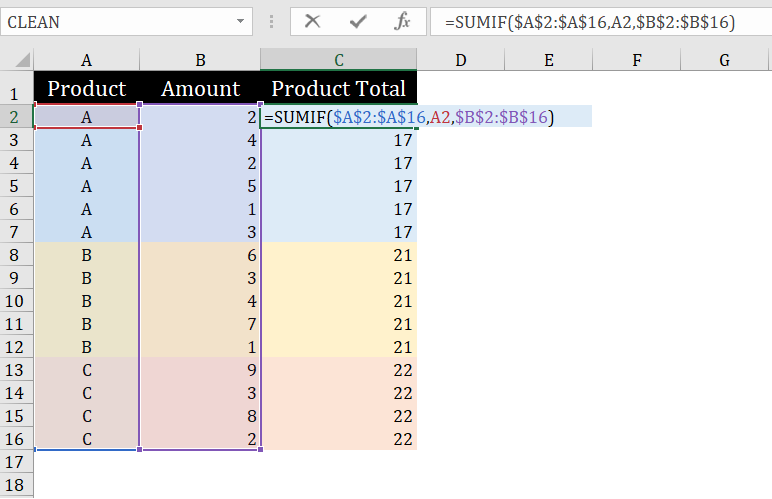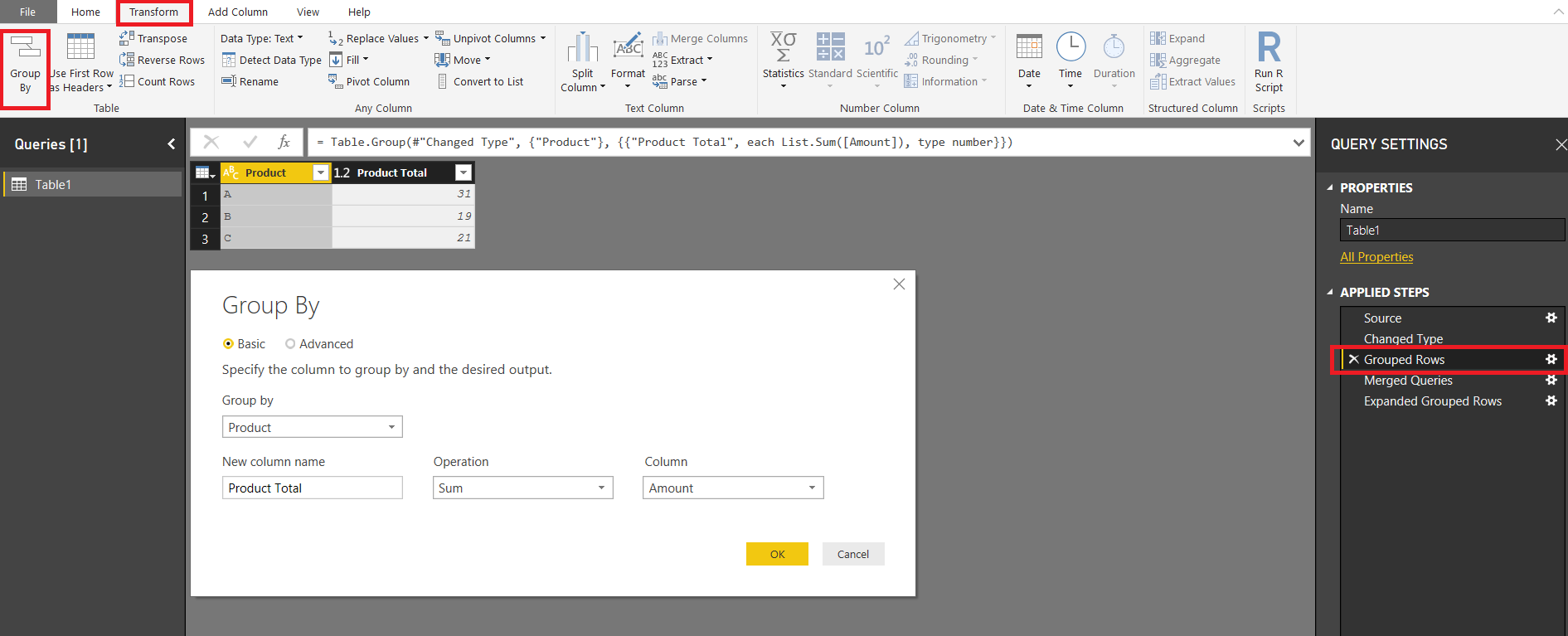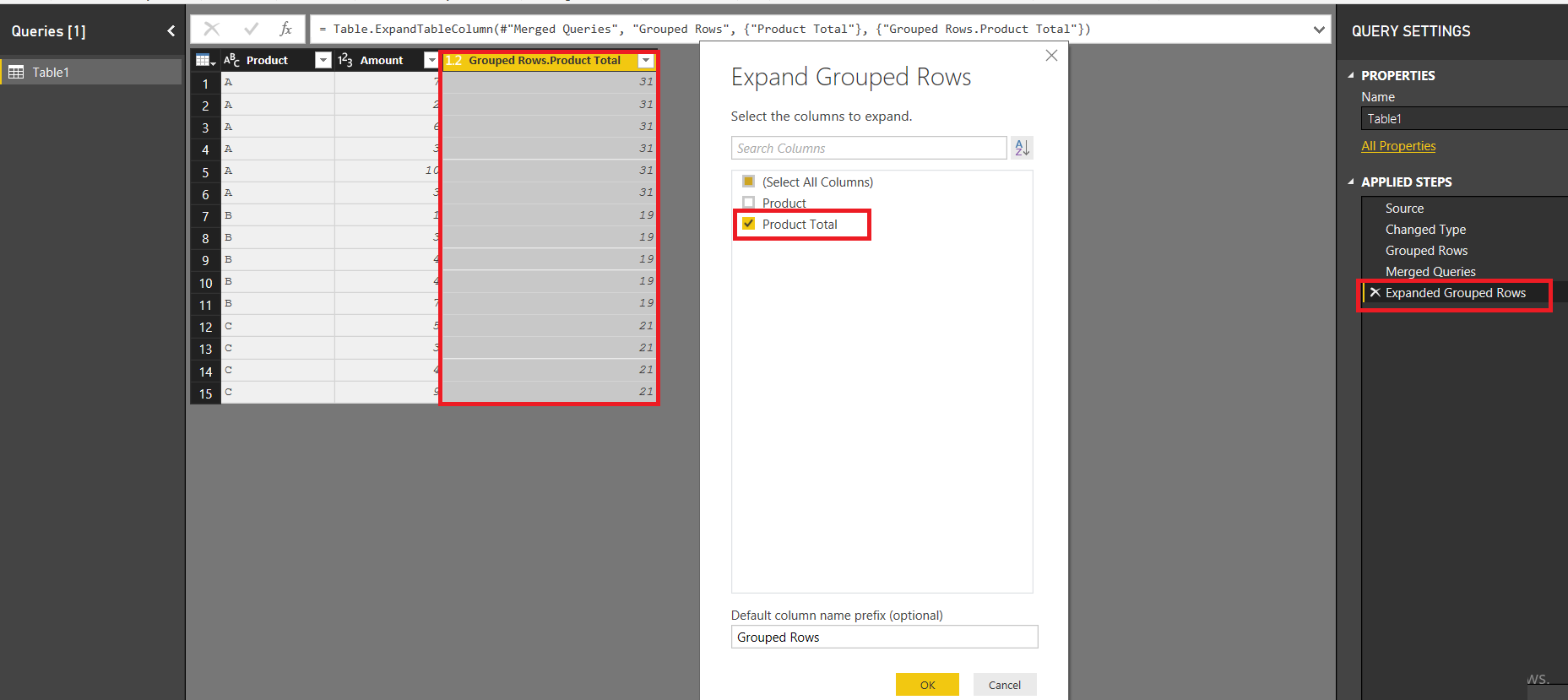# SumIf in Power Query and Calculated Column

## IN EXCEL

The SumIF in Excel is pretty easy## CALCULATED COLUMNS

USING DAX you can use one of the following formulas to achieve the desired result in a calculated column

Both these formulas essentially remove filters from Product Column before summing the Amount Column

```Product_Total =
CALCULATE ( SUM ( Table1[Amount] ), ALLEXCEPT ( Table1, Table1[Product] ) )```
```Product__Total =
CALCULATE (
SUM ( Table1[Amount] ),
FILTER ( Table1, Table1[Product] = EARLIER ( Table1[Product] ) )
)```## POWER QUERY

First Step is to do a GroupBy.. From the “Transform Tab”>>Group BYSecond Step

Now we need to find a way to merge the results of above GroupBy Operation to Original Table

But at this point the Table is already transformed…One way could be to make a copy of the Original Table and then perform the Merge

But there is a better way.. How about performing a merge of the Table before and after GroupingIf you know “M” Language, you can directly write the above code i.e.

`= Table.NestedJoin(#"Changed Type",{"Product"},#"Grouped Rows",{"Product"},"Grouped Rows",JoinKind.LeftOuter)`

Otherwise you could follow these steps as well

Go to the  Home Tab>>Merge Queries and then perform a merge of the Grouped Table with itself i.e.Now change the code generated by Query Editor…so that the merge is between the Tables before and after the Grouping i.e. GroupByThe result would beFinally you can expand the Group Rows Column to include the “Product Total” ColumnFinal “M” Code looks like this

```let
Source = Table.FromRows(Json.Document(Binary.Decompress(Binary.FromText("i45WclTSUTJXitWBsIzgLDM4yxjOMjRAE3QCCcJZCDETLCyIJc5AlimcZQxnmcBZlkqxsQA=", BinaryEncoding.Base64), Compression.Deflate)), let _t = ((type text) meta [Serialized.Text = true]) in type table [Product = _t, Amount = _t]),
#"Changed Type" = Table.TransformColumnTypes(Source,{{"Product", type text}, {"Amount", Int64.Type}}),
#"Grouped Rows" = Table.Group(#"Changed Type", {"Product"}, {{"Product Total", each List.Sum([Amount]), type number}}),
#"Merged Queries" = Table.NestedJoin(#"Changed Type",{"Product"},#"Grouped Rows",{"Product"},"Grouped Rows",JoinKind.LeftOuter),
#"Expanded Grouped Rows" = Table.ExpandTableColumn(#"Merged Queries", "Grouped Rows", {"Product Total"}, {"Grouped Rows.Product Total"})
in
#"Expanded Grouped Rows"```